Home
Hostname: page-component-55597f9d44-2qt69 Total loading time: 0.283 Render date: 2022-08-12T16:21:32.337Z Has data issue: true Feature Flags: { "shouldUseShareProductTool": true, "shouldUseHypothesis": true, "isUnsiloEnabled": true, "useRatesEcommerce": false, "useNewApi": true } hasContentIssue true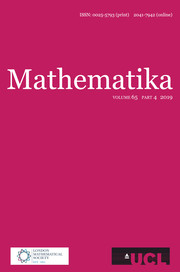Mathematika

# ON SMALL BASES FOR WHICH 1 HAS COUNTABLY MANY EXPANSIONS

Published online by Cambridge University Press:  22 January 2016

## Abstract

Let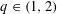$q\in (1,2)$. A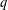$q$-expansion of a number$x$ in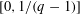$[0,1/(q-1)]$ is a sequence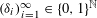$({\it\delta}_{i})_{i=1}^{\infty }\in \{0,1\}^{\mathbb{N}}$ satisfying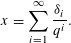$$\begin{eqnarray}x=\mathop{\sum }_{i=1}^{\infty }\frac{{\it\delta}_{i}}{q^{i}}.\end{eqnarray}$$
Let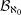${\mathcal{B}}_{\aleph _{0}}$ denote the set of$q$ for which there exists$x$ with a countable number of$q$-expansions, and let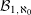${\mathcal{B}}_{1,\aleph _{0}}$ denote the set of$q$ for which$1$ has a countable number of$q$-expansions. In Erdős et al [On the uniqueness of the expansions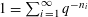$1=\sum _{i=1}^{\infty }q^{-n_{i}}$. Acta Math. Hungar.58 (1991), 333–342] it was shown that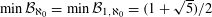$\min {\mathcal{B}}_{\aleph _{0}}=\min {\mathcal{B}}_{1,\aleph _{0}}=(1+\sqrt{5})/2$, and in S. Baker [On small bases which admit countably many expansions. J. Number Theory147 (2015), 515–532] it was shown that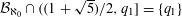${\mathcal{B}}_{\aleph _{0}}\cap ((1+\sqrt{5})/2,q_{1}]=\{q_{1}\}$, where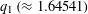$q_{1}\,({\approx}1.64541)$ is the positive root of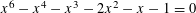$x^{6}-x^{4}-x^{3}-2x^{2}-x-1=0$. In this paper we show that the second smallest point of${\mathcal{B}}_{1,\aleph _{0}}$ is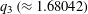$q_{3}\,({\approx}1.68042)$, the positive root of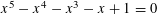$x^{5}-x^{4}-x^{3}-x+1=0$. En route to proving this result, we show that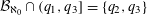${\mathcal{B}}_{\aleph _{0}}\cap (q_{1},q_{3}]=\{q_{2},q_{3}\}$, where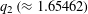$q_{2}\,({\approx}1.65462)$ is the positive root of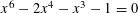$x^{6}-2x^{4}-x^{3}-1=0$.

## MSC classification

Type
Research Article
Information
Mathematika , 2016 , pp. 362 - 377

## Access options

Get access to the full version of this content by using one of the access options below. (Log in options will check for institutional or personal access. Content may require purchase if you do not have access.)

## References

Baker, S., Generalized golden ratios over integer alphabets. Integers 14 2014, Paper No. A15.Google Scholar
Baker, S., On small bases which admit countably many expansions. J. Number Theory 147 2015, 515532.CrossRefGoogle Scholar
Baker, S. and Sidorov, N., Expansions in non-integer bases: lower order revisited. Integers 14 2014, Paper No. A57.Google Scholar
Dajani, K. and Kraaikamp, C., Random 𝛽-expansions. Ergodic Theory Dynam. Systems 23 2003, 461479.CrossRefGoogle Scholar
Dajani, K. and de Vries, M., Measures of maximal entropy for random 𝛽-expansions. J. Eur. Math. Soc. 7 2005, 5168.CrossRefGoogle Scholar
Dajani, K. and de Vries, M., Invariant densities for random 𝛽-expansions. J. Eur. Math. Soc. 9 2007, 157176.CrossRefGoogle Scholar
Daróczy, Z. and Kátai, I., Univoque sequences. Publ. Math. Debrecen 42 1993, 397407.Google Scholar
Daróczy, Z. and Kátai, I., On the structure of univoque numbers. Publ. Math. Debrecen 46 1995, 385408.Google Scholar
Erdős, P., Horváth, M. and Joó, I., On the uniqueness of the expansions 1 =∑ i=1 q n i . Acta Math. Hungar. 58 1991, 333342.Google Scholar
Erdős, P. and Joó, I., On the number of expansions 1 =∑ q n i . Ann. Univ. Sci. Budapest. Eötvös Sect. Math. 35 1992, 129132.Google Scholar
Erdős, P. and Joó, I., On the number of expansions 1 =∑ q n i , II. Ann. Univ. Sci. Budapest. Eötvös Sect. Math. 36 1993, 229233.Google Scholar
Erdős, P., Joó, I. and Komornik, V., Characterization of the unique expansions 1 =∑ i=1 q n i and related problems. Bull. Soc. Math. France 118 1990, 377390.CrossRefGoogle Scholar
Glendinning, P. and Sidorov, N., Unique representations of real numbers in non-integer bases. Math. Res. Lett. 8 2001, 535543.CrossRefGoogle Scholar
Komornik, V. and Loreti, P., Unique developments in non-integer bases. Amer. Math. Monthly 105 1998, 636639.CrossRefGoogle Scholar
Parry, W., On the 𝛽-expansions of real numbers. Acta Math. Acad. Sci. Hungar. 11 1960, 401416.CrossRefGoogle Scholar
Sidorov, N., Almost every number has a continuum of 𝛽-expansions. Amer. Math. Monthly 110 2003, 838842.Google Scholar
Sidorov, N., Ergodic-theoretic properties of certain Bernoulli convolutions. Acta Math. Hungar. 101 2003, 345355.CrossRefGoogle Scholar
Sidorov, N., Expansions in non-integer bases: lower, middle and top orders. J. Number Theory 129 2009, 741754.CrossRefGoogle Scholar
de Vries, M. and Komornik, V., Unique expansions of real numbers. Adv. Math. 221 2009, 390427.CrossRefGoogle Scholar
Zou, Y. R., Lu, J. and Li, W. X., Unique expansion of points of a class of self-similar sets with overlaps. Mathematika 58 2012, 371–388.CrossRefGoogle Scholar
2
Cited by

# Save article to Kindle

Note you can select to save to either the @free.kindle.com or @kindle.com variations. ‘@free.kindle.com’ emails are free but can only be saved to your device when it is connected to wi-fi. ‘@kindle.com’ emails can be delivered even when you are not connected to wi-fi, but note that service fees apply.

Find out more about the Kindle Personal Document Service.

ON SMALL BASES FOR WHICH 1 HAS COUNTABLY MANY EXPANSIONS
Available formats
×

# Save article to Dropbox

To save this article to your Dropbox account, please select one or more formats and confirm that you agree to abide by our usage policies. If this is the first time you used this feature, you will be asked to authorise Cambridge Core to connect with your Dropbox account. Find out more about saving content to Dropbox.

ON SMALL BASES FOR WHICH 1 HAS COUNTABLY MANY EXPANSIONS
Available formats
×

# Save article to Google Drive

To save this article to your Google Drive account, please select one or more formats and confirm that you agree to abide by our usage policies. If this is the first time you used this feature, you will be asked to authorise Cambridge Core to connect with your Google Drive account. Find out more about saving content to Google Drive.

ON SMALL BASES FOR WHICH 1 HAS COUNTABLY MANY EXPANSIONS
Available formats
×
×